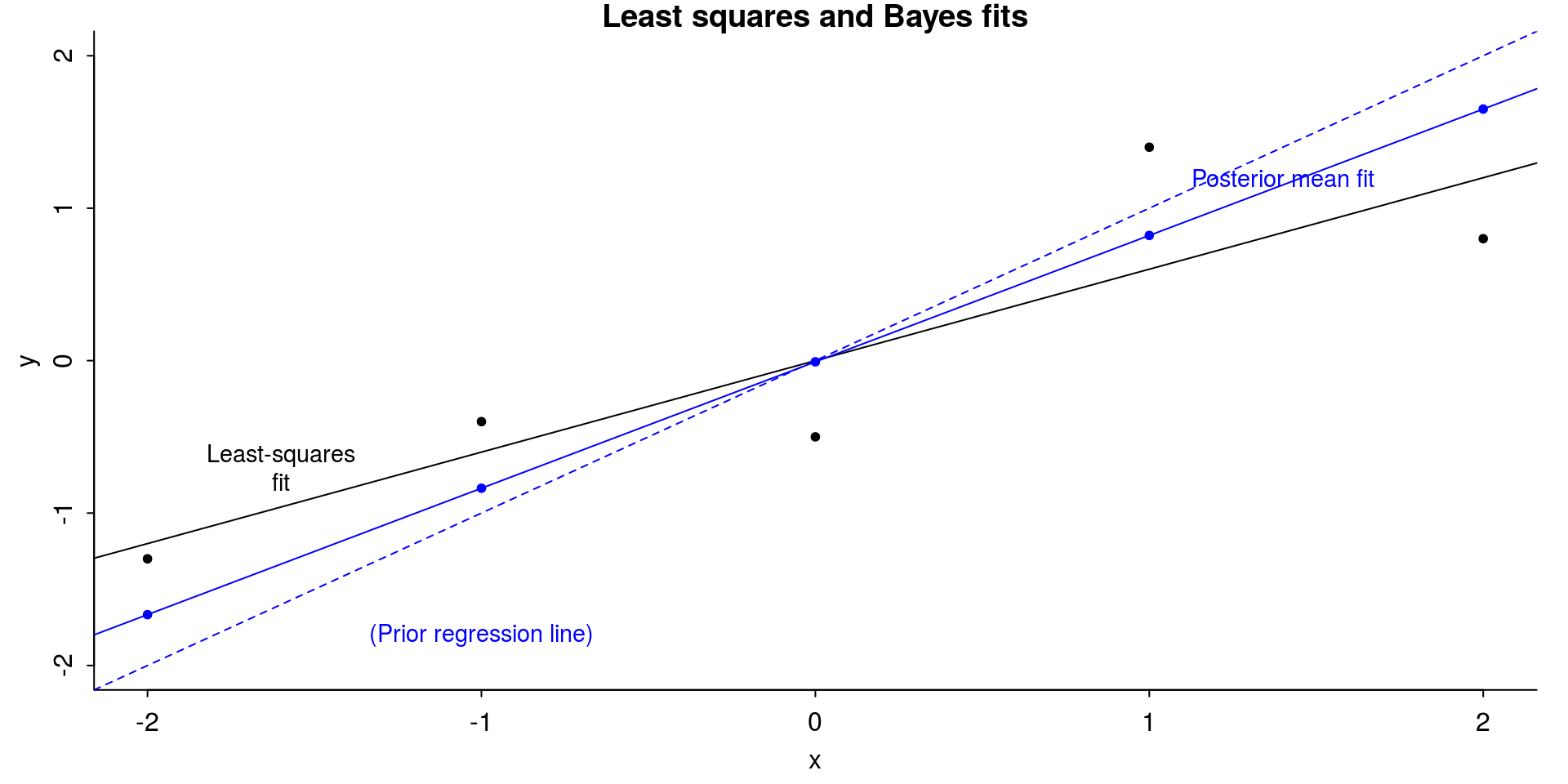# 1 Introduction

This notebook demonstrates Bayesian posterior distributions of model based R-squared and LOO-R2 measures for linear and logistic regression. This notebook is a supplement for the paper

We specifically define R-squared as $R^2 = \frac{{\mathrm Var}_{\mu}}{{\mathrm Var}_{\mu}+{\mathrm Var}_{\mathrm res}},$ where $${\mathrm Var}_{\mu}$$ is variance of modelled predictive means, and $${\mathrm Var}_{\mathrm res}$$ is the modelled residual variance. Specifically both of these are computed only using posterior quantities from the fitted model.

The residual based R2 uses draws from the residual distribution and is defined as ${\mathrm Var}_{\mu}^s = V_{n=1}^N \hat{y}_n^s\\ {\mathrm Var}_{\mathrm res}^s = V_{n=1}^N \hat{e}_n^s,$ where $$\hat{e}_n^s=y_n-\hat{y}_n^s$$.

The model based R2 uses draws from the modeled residual variances. For linear regression we define ${\mathrm Var}_{\mathrm res}^s = (\sigma^2)^s,$ and for logistic regression, following Tjur (2009), we define ${\mathrm Var}_{\mathrm res}^s = \frac{1}{N}\sum_{n=1}^N (\pi_n^s(1-\pi_n^s)),$ where $$\pi_n^s$$ are predicted probabilities.

The LOO-R2 uses LOO residuals and is defined $$1-{\mathrm Var}_{\mathrm loo-res}/{\mathrm Var}_{y}$$ and ${\mathrm Var}_{y} = V_{n=1}^N {y}_n\\ {\mathrm Var}_{\mathrm loo-res} = V_{n=1}^N \hat{e}_{{\mathrm loo},n},$ where $$\hat{e}_{{\mathrm loo},n}=y_n-\hat{y}_{{\mathrm loo},n}$$. The uncertainty in LOO-R2 comes from not knowing the future data distribution (Vehtari and Ojanen, 2012). We can approximate draws from the corresponding distribution using Bayesian bootstrap (Vehtari and Lampinen, 2002).

File contents:

• bayes_R2_res function (using residuals)
• bayes_R2 function (using sigma and latent space for binary)
• loo_R2 function (using LOO residuals and Bayesian bootstrap for uncertainty)
• code for fitting the models for the example in the paper
• code for producing the plots in the paper (both base graphics and ggplot2 code)
• several examples

Corresponding Bayes_R2.R file

library("rprojroot")
root<-has_dirname("RAOS-Examples")$make_fix_file() library("rstanarm") options(mc.cores = parallel::detectCores()) library("loo") library("ggplot2") library("bayesplot") theme_set(bayesplot::theme_default(base_family = "sans")) library("latex2exp") library("foreign") library("bayesboot") SEED <- 1800 set.seed(SEED) # 2 Functions for Bayesian R-squared for stan_glm models. A more complicated version of this function that is compatible with stan_glmer models and specifying newdata will be available in the rstanarm package. @param fit A fitted model object returned by stan_glm. @return A vector of R-squared values with length equal to the number of posterior draws. Bayes-R2 function using residuals bayes_R2_res <- function(fit) { y <- rstanarm::get_y(fit) ypred <- rstanarm::posterior_linpred(fit, transform = TRUE) if (family(fit)$family == "binomial" && NCOL(y) == 2) {
trials <- rowSums(y)
y <- y[, 1]
ypred <- ypred %*% diag(trials)
}
e <- -1 * sweep(ypred, 2, y)
var_ypred <- apply(ypred, 1, var)
var_e <- apply(e, 1, var)
var_ypred / (var_ypred + var_e)
}

Bayes-R2 function using modelled (approximate) residual variance

bayes_R2 <- function(fit) {
mupred <- rstanarm::posterior_linpred(fit, transform = TRUE)
var_mupred <- apply(mupred, 1, var)
if (family(fit)$family == "binomial" && NCOL(y) == 1) { sigma2 <- apply(mupred*(1-mupred), 1, mean) } else { sigma2 <- as.matrix(fit, pars = c("sigma"))^2 } var_mupred / (var_mupred + sigma2) } LOO-R2 function using LOO-residuals and Bayesian bootstrap loo_R2 <- function(fit) { y <- get_y(fit) ypred <- posterior_linpred(fit, transform = TRUE) ll <- log_lik(fit) M <- length(fit$stanfit@sim$n_save) N <- fit$stanfit@sim$n_save[] r_eff <- relative_eff(exp(ll), chain_id = rep(1:M, each = N)) psis_object <- psis(log_ratios = -ll, r_eff = r_eff) ypredloo <- E_loo(ypred, psis_object, log_ratios = -ll)$value
eloo <- ypredloo-y
n <- length(y)
rd<-rudirichlet(4000, n)
vary <- (rowSums(sweep(rd, 2, y^2, FUN = "*")) -
rowSums(sweep(rd, 2, y, FUN = "*"))^2)*(n/(n-1))
vareloo <- (rowSums(sweep(rd, 2, eloo^2, FUN = "*")) -
rowSums(sweep(rd, 2, eloo, FUN = "*")^2))*(n/(n-1))
looR2 <- 1-vareloo/vary
looR2[looR2 < -1] <- -1
looR2[looR2 > 1] <- 1
return(looR2)
}

# 3 Experiments

## 3.1 Toy data with n=5

x <- 1:5 - 3
y <- c(1.7, 2.6, 2.5, 4.4, 3.8) - 3
xy <- data.frame(x,y)

Lsq fit

fit <- lm(y ~ x, data = xy)
ols_coef <- coef(fit)
yhat <- ols_coef + ols_coef * x
r <- y - yhat
rsq_1 <- var(yhat)/(var(y))
rsq_2 <- var(yhat)/(var(yhat) + var(r))
round(c(rsq_1, rsq_2), 3)
 0.766 0.766

Bayes fit

fit_bayes <- stan_glm(y ~ x, data = xy,
prior_intercept = normal(0, 0.2, autoscale = FALSE),
prior = normal(1, 0.2, autoscale = FALSE),
prior_aux = NULL,
seed = SEED
)
posterior <- as.matrix(fit_bayes, pars = c("(Intercept)", "x"))
post_means <- colMeans(posterior)

Median Bayesian R2

round(median(bayesR2res<-bayes_R2_res(fit_bayes)), 2)
 0.8
round(median(bayesR2<-bayes_R2(fit_bayes)), 2)
 0.75

LOO R2

looR2<-loo_R2(fit_bayes)
Warning: Some Pareto k diagnostic values are too high. See help('pareto-k-diagnostic') for details.
round(median(looR2), 2)
 0.57

With small n LOO-R2 is much lower than median of Bayesian R2

Figures

The first section of code below creates plots using base R graphics.
Below that there is code to produce the plots using ggplot2.

# take a sample of 20 posterior draws
keep <- sample(nrow(posterior), 20)
samp_20_draws <- posterior[keep, ]

Base graphics version

par(mar=c(3,3,1,1), mgp=c(1.7,.5,0), tck=-.01)
plot(
x, y,
ylim = range(x),
xlab = "x",
ylab = "y",
main = "Least squares and Bayes fits",
bty = "l",
pch = 20
)
abline(coef(fit), coef(fit), col = "black")
text(-1.6,-.7, "Least-squares\nfit", cex = .9)
abline(0, 1, col = "blue", lty = 2)
text(-1, -1.8, "(Prior regression line)", col = "blue", cex = .9)
abline(coef(fit_bayes), coef(fit_bayes), col = "blue")
text(1.4, 1.2, "Posterior mean fit", col = "blue", cex = .9)
points(
x,
coef(fit_bayes) + coef(fit_bayes) * x,
pch = 20,
col = "blue"
)par(mar=c(3,3,1,1), mgp=c(1.7,.5,0), tck=-.01)
plot(
x, y,
ylim = range(x),
xlab = "x",
ylab = "y",
bty = "l",
pch = 20,
main = "Bayes posterior simulations"
)
for (s in 1:nrow(samp_20_draws)) {
abline(samp_20_draws[s, 1], samp_20_draws[s, 2], col = "#9497eb")
}
abline(
coef(fit_bayes),
coef(fit_bayes),
col = "#1c35c4",
lwd = 2
)
points(x, y, pch = 20, col = "black")